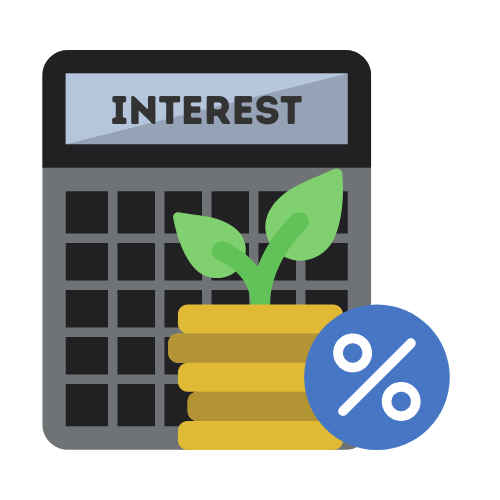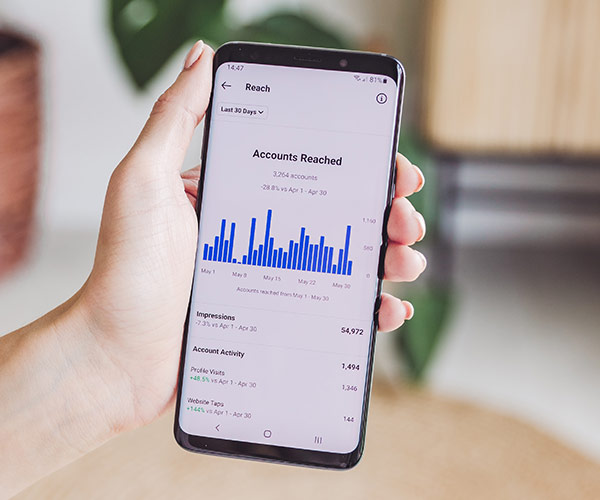# Compound Interest Calculator

## Calculate compound interest on your savings and investments today

Enter a number.
Enter a number.
Enter a number.
Enter a number.

Total Savings

Available at

View the savings breakdown

## What is Compound Interest?

Albert Einstein famously called it the 8th wonder of the world.

Compound interest is one of the most powerful forces in the universe, which is why you need to understand how it works if you want to have great returns on your investment. Compound interest can also be called reinvesting interest, which means if you have money in the bank and you continue to get interest on it, that interest is added to your principal, meaning at the end of the year, you will have more money.

Savings Breakdown
Year Principal Savings

## Why use a financial calculator?

Using a financial calculator like a compound interest calculator or savings calculator makes it easier for you to manage your finances. Compounding interest can be very effective over longer durations.

Calculating compound interest using a basic calculator is not easy as the interest amounts need to be invested back into the principle and further interest is calculated on the new amount at each interval. Therefore using an online calculator is a great option to simplify the process.

## Why is compound interest important?

It is probably something you have never thought about, but compound interest is actually a pretty interesting concept that goes a lot deeper than simply having interest on your interest. The idea is that when money is left to grow on its own, it will do so at a rate that is faster than the original interest rate.

This is because the interest rate is also added to the principal, and the interest is then applied to the new principal, which then earns interest and so on. In fact, the concept of compound interest is so interesting and useful, that it is even used in everything from physics to computer science.

## How compound interest works

Compound interest is a phenomenon of earning interest on your interest. In other words, you earn interest on money you already have, rather than just on the original principal amount. Compound interest - formula-ically speaking -- is pretty simple.

You just take the principal amount plus the accumulated interest and then earn interest on that total. As time goes on, the amount of interest you earn builds and builds. This means that your money grows at a faster and faster rate.## Benefits of using a compound interest calculator

Financial calculators like those calculating savings or compound interest, greatly simplify your finances. They allow you to add the key variables and quickly get presented with financial outcomes based on the values you enter.

One of the great things about this tool is that it makes it easy to see how much of the total financial value of your investment has been created from compounding interest and how much is from the original principle payments.

## Who invented compound interest?

The legendary Albert Einstein once said: "Compound interest is the eighth wonder of the world. He who understands it, earns it - he who doesn't - pays it." The truth of this statement has been proven time and again. Compound interest is a bit like magic and it has the power to change your life - for the better.

Compound interest is thought to be first used in Italy, sometime during the 17th centuary. Since then it has become widely adopted and applies to many different financial investment accounts.

## How does Compound interest work?

Compound interest can be described as "interest on interest". It is the concept of calculating interest on both the principal investment and previous interest. The new interest is earned on the old interest and this process repeats itself. The concept of compound interest is very important in finance and economics. It is the rate of interest that is earned not only on the principal invested, but also on the interest already earned, so that interest is earned on interest.

## Where is compound interest used?

Compound interest is a tool for making your money work harder for you. It is the process of earning interest on money that has already been earning interest. When you invest into a savings account, you are earning interest on the additional money that you have invested, plus you will also earn interest on the money that was already in the account and accruing interest each month.

The interest then gets added to the total balance, and on the next interval the profit will be calculated from the initial investments along with the profit added. Compound interest is a powerful method for growing your money and is often used by banks for investing. With compound interest, even small amounts of money can earn large sums of money.

## Can compound interest be negative?

Compound interest can be negative. If you have debts that continue to grow with the interest charges, the interest can grow large enough that it will end up surpassing your original amount. You can also have a negative compound interest with a failing business that may not be able to pay back the loans into which it is required to pay interest. This is not good for the business and will likely lead to its collapse.

## Can compound interest make you rich?

Compound interest is a financial tool widely used in modern financial institutions. It is a mechanism for generating growth in a savings account. It is most often applied in a situation when an investor puts money into a savings account or a similar product and the bank or other financial institution adds interest to the account.

The interest is then added to the account balance so that the next time the account is credited with interest, the new amount is added to the balance and the interest is again added to the new amount and so on. The longer the period of time the money is left in the account, the higher the account balance becomes because of the accumulation of interest. Compound interest is the reason why some people are rich while others are poor.

## How to use compound interest calculator with contributions

The financial calculator is a useful tool used to see how much money you can save in the future. It's very useful when used correctly with a bit of financial planning for the future. Compound interest is very powerful but it can also be a double-edged sword if not used correctly.

Once you know how it works, you can use it to your advantage. The free calculator on this page will allow you to account for regular investments or contributions to your account and include those into the total balance after compounding interest.

## Is simple or compound interest better?

The word "interest" has several meanings, some of which are ambiguous and confusing. In the context of finance, however, interest refers to the fee charged by a lender for the use of a sum of money. There are two main types of interest used in the financial world: simple interest and compound interest.

Simple interest is charged on the principal amount and does not take into account the money earned from interest. Compound interest is charged on the initial principal plus all the interest that has added up over time. Compound interest is a more effective way to grow your savings because of its ability to multiply the effects of interest. Compound interest is different from simple interest.

The advantages compound interest has over simple interest are too significant to ignore. Compound interest is an investment tool that can help you earn more money than you think.## Difference between simple interest and compound interest

Compound interest is different from simple interest in the sense that it is earned not only from the principal but also on the interest that is accumulated. Compound interest is applied when you need a higher principal amount or a larger sum of money in the future. This type of interest is taken into consideration by banks when a business or an individual applies for a loan.

Compound interest is an important factor that we need to consider in order to make the most out of our money. Now the question is, how do we calculate compound interest? Actually, there are a lot of different ways on how to compute compound interest, either manually or by using a computer.

## Is compound interest calculated on a monthly or yearly basis?

For most people, compounding interest is normally calculated on a monthly basis. However, this is not always the case. There are some situations in which the interest may be calculated on different interval.

Banks and credit unions may set the compound at quarterly or even semiannually, but monthly is the default for most institutions. The interval in which the interest is calculated will vary between different financial institutions and the investment products that they offer consumers so it is always good to check with your bank, financial organisation or lending institution before committing to investing your savings there.